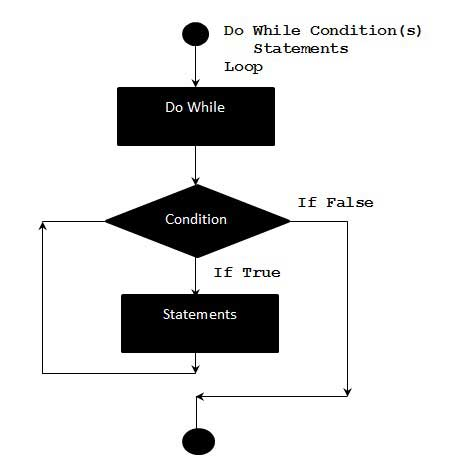# VBScript Do..While statement

A Do..While loop is used when we want to repeat a set of statements as long as the condition is true. The Condition may be checked at the beginning of the loop or at the end of the loop.

## Syntax

The syntax of a Do..While loop in VBScript is −

```Do While condition
[statement 1]
[statement 2]
...
[statement n]
[Exit Do]
[statement 1]
[statement 2]
...
[statement n]
Loop
```

## Flow Diagram## Example

The below example uses Do..while loop to check the condition at the beginning of the loop. The statements inside the loop are executed only if the condition becomes True.

```<!DOCTYPE html>
<html>
<body>
<script language = "vbscript" type = "text/vbscript">
Do While i < 5
i = i + 1
Document.write("The value of i is : " & i)
Document.write("<br></br>")
Loop
</script>
</body>
</html>
```

When the above code is executed, it prints the following output on the console.

```The value of i is : 1

The value of i is : 2

The value of i is : 3

The value of i is : 4

The value of i is : 5
```

## Alternate Syntax

There is also an alternate Syntax for Do..while loop which checks the condition at the end of the loop. The Major difference between these two syntax is explained below with an example.

```Do
[statement 1]
[statement 2]
...
[statement n]
[Exit Do]
[statement 1]
[statement 2]
...
[statement n]
Loop While condition
```

## Flow Diagram## Example

The below example uses Do..while loop to check the condition at the end of the loop. The Statements inside the loop are executed atleast once even if the condition is False.

```<!DOCTYPE html>
<html>
<body>
<script language = "vbscript" type = "text/vbscript">
i = 10
Do
i = i + 1
Document.write("The value of i is : " & i)
Document.write("<br></br>")
Loop While i<3 'Condition is false.Hence loop is executed once.

</script>
</body>
</html>
```

When the above code is executed, it prints the following output in the console.

```The value of i is : 11
```
vbscript_loops.htm